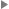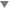## informalequation

informalequation — A displayed mathematical equation without a title.

## Description

An `informalequation` is usually a mathematical equation or a group of related mathematical equations.

### Processing expectations

Formatted as a displayed block.

#### ParentsThis element occurs in 73 elements.

### Children

The following elements occur in informalequation: `alt`, `caption` (db.caption), `info` (db.titleforbidden.info), `mathphrase`, `mediaobject`, `mml:*`.

## Examples

```  1 <article xmlns='http://docbook.org/ns/docbook'>
2 <title>Example informalequation</title>

4 <para>The equation
<informalequation>
6   <alt>e^(pi*i) + 1 = 0</alt>
<mediaobject>
8     <imageobject condition="print">
<imagedata fileref="figs/print/db5d_refeqn02.pdf"/>
10     </imageobject>
<imageobject condition="web">
12       <imagedata fileref="figs/web/db5d_refeqn02.png"/>
</imageobject>
14   </mediaobject>
</informalequation>
16 is delightful because it joins together five of the most
important mathematical constants.
18 </para>

20 </article>```

The equation

is delightful because it joins together five of the most important mathematical constants.

Last revised by Norman Walsh on 6 Jun 2011 (git hash: 6ffcc7640bbc5f852a318e452c9f210f03292cb9)